#Function Repository Resource:

# CotDegree

Compute the cotangent of an angle given in degrees

Contributed by: Paco Jain (Wolfram Research)
 ResourceFunction["CotDegree"][x] computes the cotangent of an angle x, measured in degrees.

## Details and Options

CotDegree is a mathematical function, suitable for both symbolic and numerical manipulation.
For certain special arguments, CotDegree automatically evaluates to exact values.
CotDegree can be evaluated to arbitrary numerical precision.
CotDegree automatically threads over lists.

## Examples

### Basic Examples

Compute the cotangent of an angle:

 In:=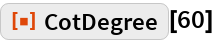Out=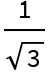Plot over a given range of angles:

 In:=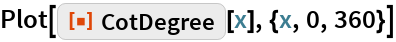Out=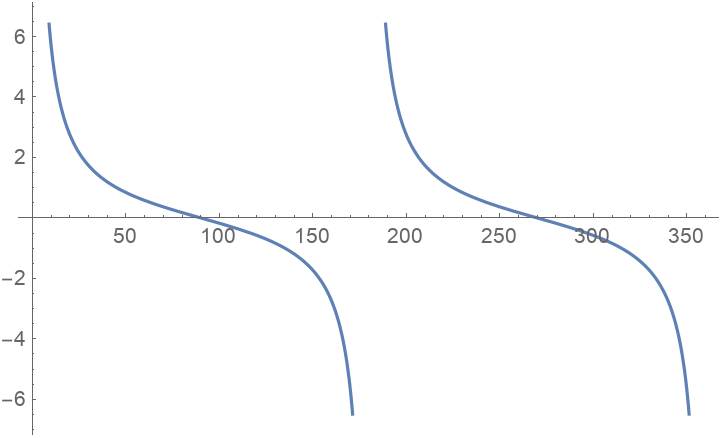### Scope

Evaluate numerically:

 In:=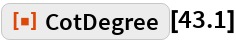Out=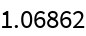Evaluate to high precision:

 In:=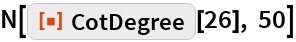Out=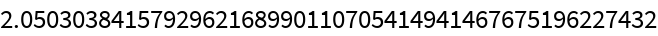## Requirements

Wolfram Language 11.3 (March 2018) or above